# What accelerates the potential

## Electric potential and voltage

### Potential and voltage in the electric field

There is a charged body with the charge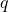in a homogeneous electric field of a plate capacitor, the constant force acts on it at every location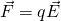.

This force can accelerate it in a homogeneous field.

In doing so, he takes the energy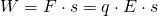where s is the distance over which it was accelerated.

He then owns the kinetic energy.

Assuming that the body is positively charged and is in the middle of the capacitor - it can then be accelerated over a distance that corresponds to half the distance d.

He can use the energy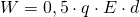or.take up.

In other words:

He has that potential, the energy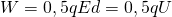to record.

If the body is on the positively charged plate, it can be accelerated over the entire distance d. So he has that potential, the energy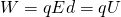to record.

If, on the other hand, it is on the negative plate (Point p0), it can no longer absorb energy.

The amount of energy that the charged body can absorb depends on the place, of the Voltage U and its charge q.

So for each point of the field a certain energy and thus a certain value for the quotient can be set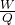assign.

This quotient is called electrical potential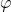compared to point P0 denotes and indicates which energy a certain charge q can absorb in the field or which energy is needed to transfer this charge from point P.0 to a point Pi bring to.